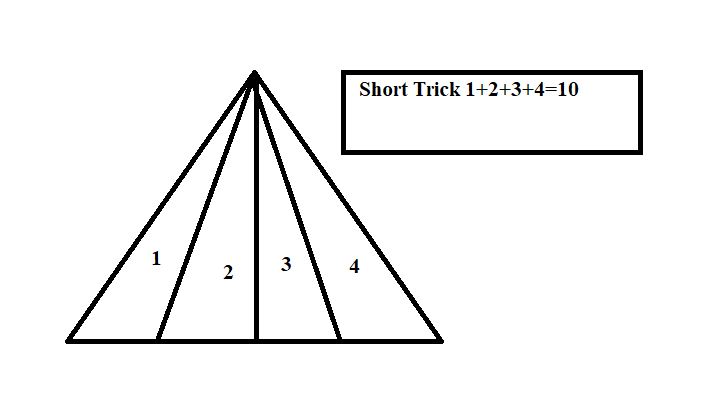• Save

# Figure Counting Questions with Answers for Competitive Exams

6 months ago 29.3K Views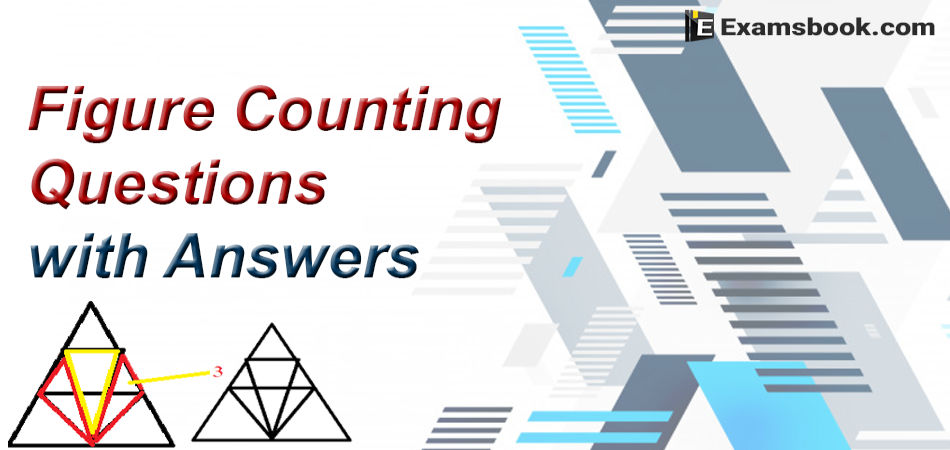Are you confused in preparing figure counting questions with answers in the competitive exams? Don't worry! here you can get easily figure counting questions with answers for your preparation. Here are given the important figure counting questions with the explanation which will help you to cover the reasoning section and help to improve your score in the various competitive exams.

## Selective Figure Counting Questions

Q :

निम्नलिखित प्रश्न में त्रिभुज हैं

(A) 21

(B) 23

(C) 25

(D) 27

Explanation :

The main triangle shown is in the given figure and this the total no. of triangle is 15. remaing triangle we can find out in the drawing the triangle in the image.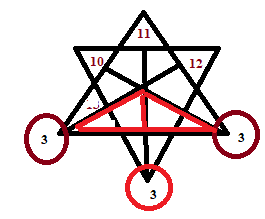Q :

Find the number of triangle in the given figure.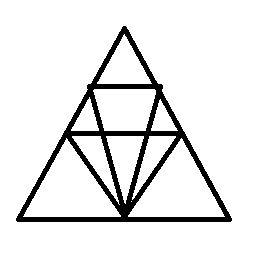(A) 12

(B) 18

(C) 22

(D) 26

Explanation :

The total number of triangle is according to figure is 2+3+3+2+8=18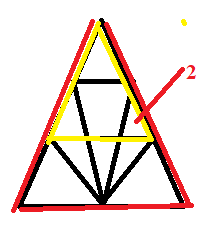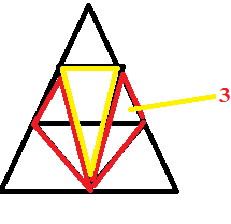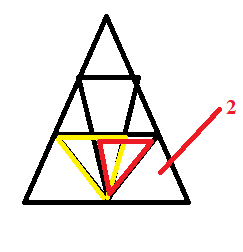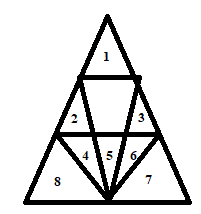Q :

In the question find the number of triangles.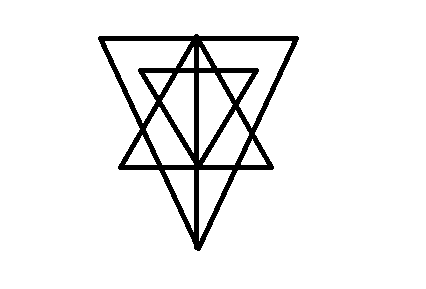(A) 27

(B) 25

(C) 23

(D) 21

Explanation :

In the figure the simplest Triangles are GLK, DLJ, DJM,HMN,QRE,IRA,IPA, and FPO is the 8 triangle

and another is BDO,CDQ,DLM, PRA, KFI, NEI, HJI, GJI,DKI, and DNI is the 10 triangle

and another is DIE, DFI, DOA, DQA, and GHI is the 5 triangle

and DCA, DBA is the 2 triangle and DEF and ABC so that according to the figure the total no. of triangle is 8+10+5+2+1+1=27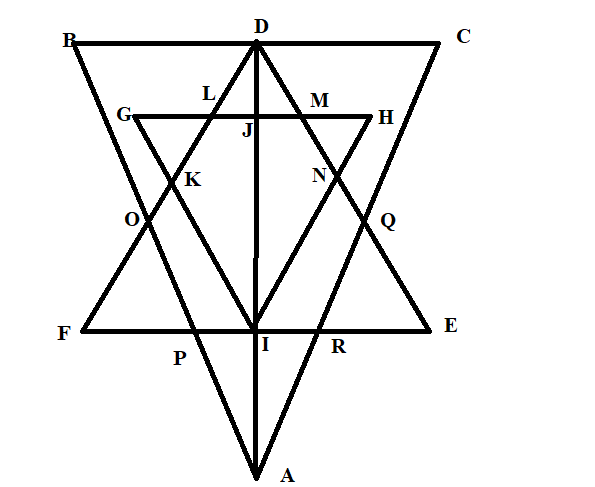Q :

In question below shown, count the number of triangle and squares.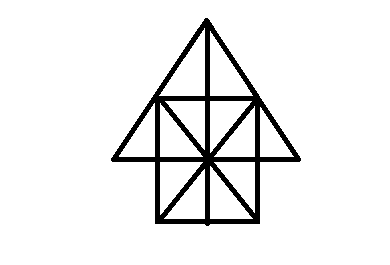(A) 26 triangles, 5 squares

(B) 28 triangles, 5 squares

(C) 26 triangles,6 squares

(D) 28 triangles, 6 squares

Explanation :

The simplest triangle is shown in figure is 12 and another is IBO, BDO, DGO, GIO, ABO,CBD, DEO, IBD,BDG, DGI, GIB, ACO and COE and ACE is the total no of traingle are 12+14=26

Q :

In the following questions, count the number of triangles and squares in the given figure.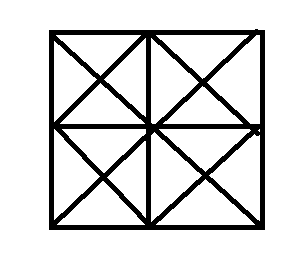(A) 44 triangles,10 squares

(B) 14 triangles, 16 squares

(C) 27 triangles,6 squares

(D) 36 triangles, 9 squares

Q :

दी गई  आकृति में कितने त्रिभुज है?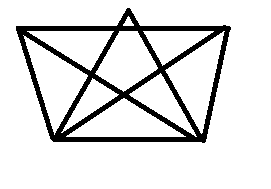(A) 16

(B) 19

(C) 10

(D) 25

Q :

Find the number of triangles in the figure.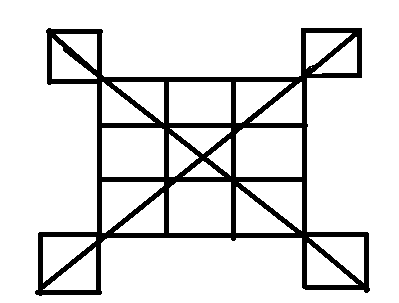(A) 20

(B) 24

(C) 28

(D) 32

Explanation :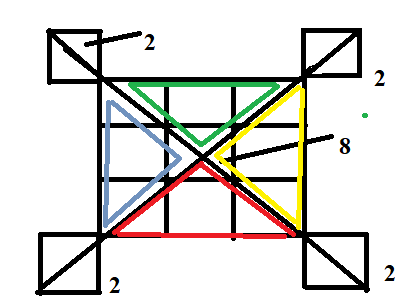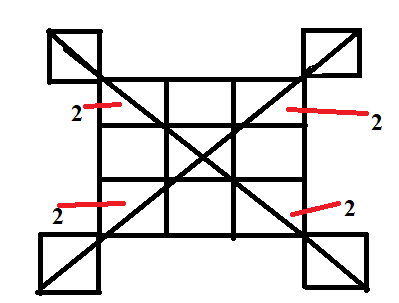The figure shown the no. of triangle is in the diagram so that total no. of triangle is 8+8+8+4=28

Q :

How many triangles in the figure?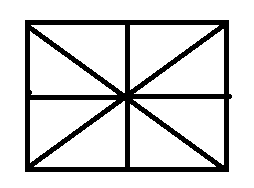(A) 16

(B) 14

(C) 8

(D) 12

Explanation :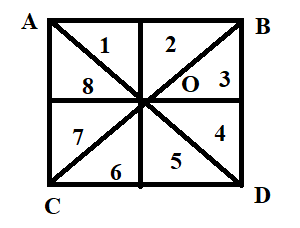in the figure the 8 triangle is shown easily and another is COD , DOB, BOA, AOC, ACD, BDC,ABC, ABD so that total no. of triangle is 8+8=16

Q :

How many squares in the figure?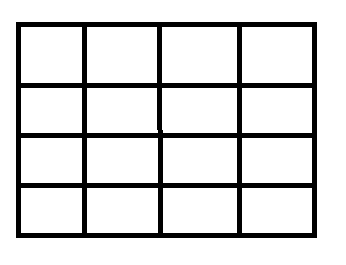(A) 16

(B) 17

(C) 26

(D) 30

Explanation :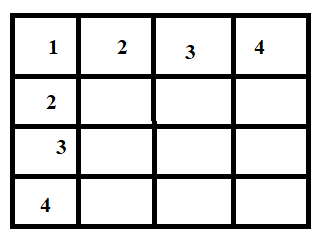The total squares is in the give figure is 12+22+32+42=30

Q :

How many triangle is in the given figure?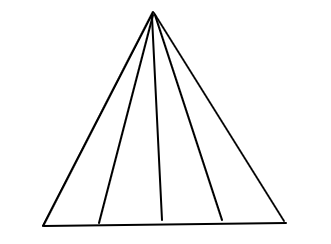(A) 10

(B) 12

(C) 14

(D) 16

Explanation :